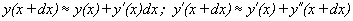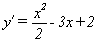Chapter IV.E  Problems 23,25,27
Solutions.

Key for estimations:#23: dx =1

 x y y' y'dx = dy y'' = x-3 y'' dx 0 5 2 2 -3 -3 1 7 -1 -1 -2 -2 2 6 -3 -3 -1 -1 3 3 -4 -4 0 0 4 -1 -4 -4 1 1

The exact solution for this problem isand y = x 3/6  - 3/2 x2 + 2x + 5 .
Thus y(4)= 64/6 -48/2 +8 +5 = -2/3.

In fact y(3) = 27/6 -27/2 + 6 +5 = 2. Since y'' < 0 for x < 3, the solution function has a graph that is concave down for x <3, so the differential estimator used in Euler's method is an overestimate. However, the solution is concave up for 3 < x < 4,and so the estimate can change, as it does, to an underestimate despite the accumulated error in the estimation of y(3).

25: dx = 1

 x y y' y'dx = dy y'' = -y y'' dx 0 0 1 1 0 0 1 1 1 1 -1 -1 2 2 0 0 -2 -2 3 2 -2 -2 -2 -2 4 0 -4 -4 0 0

27: dx =1/2

 x y y' y'dx = dy y'' = y y'' dx 0.00 5.00 2.00 1.00 5.00 2.50 0.50 6.00 4.50 2.25 6.00 3.00 1.00 8.25 7.50 3.75 8.25 4.13 1.50 12.00 11.63 5.81 12.00 6.00 2.00 17.81 17.63 8.81 17.81 8.91 2.50 26.63 26.53 13.27 26.63 13.31 3.00 39.89 39.84 19.92 39.89 19.95 3.50 59.81 59.79 29.89 59.81 29.91 4.00 89.71 89.70 44.85 89.71 44.85